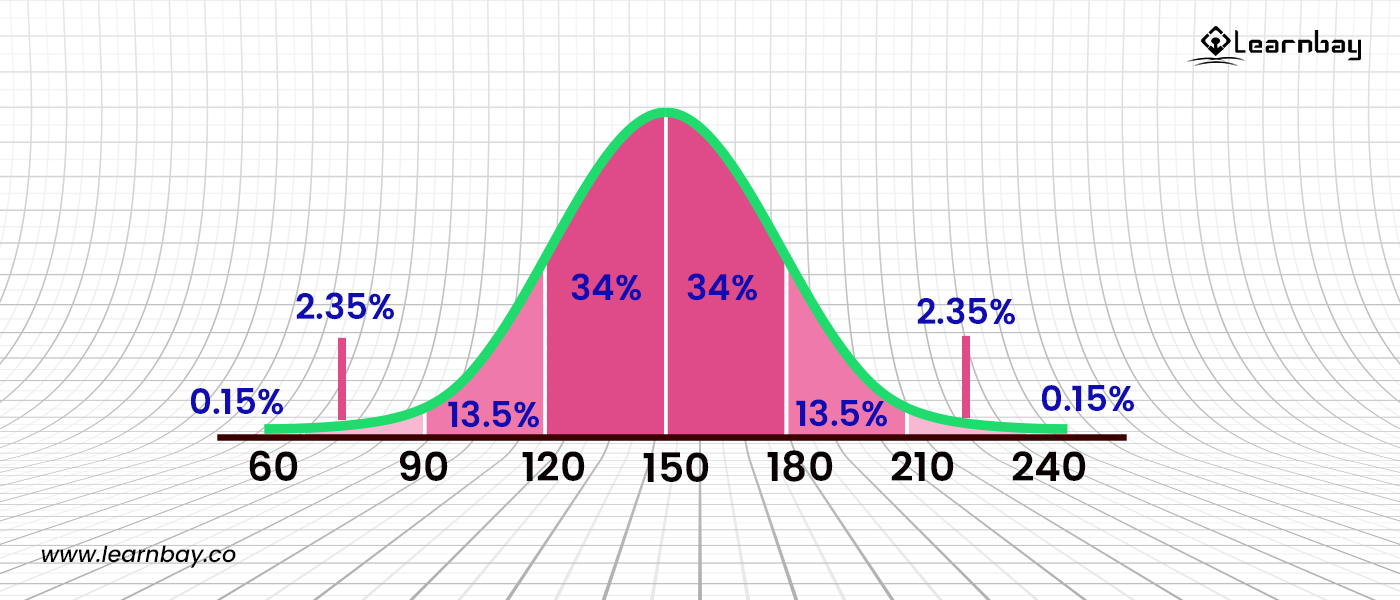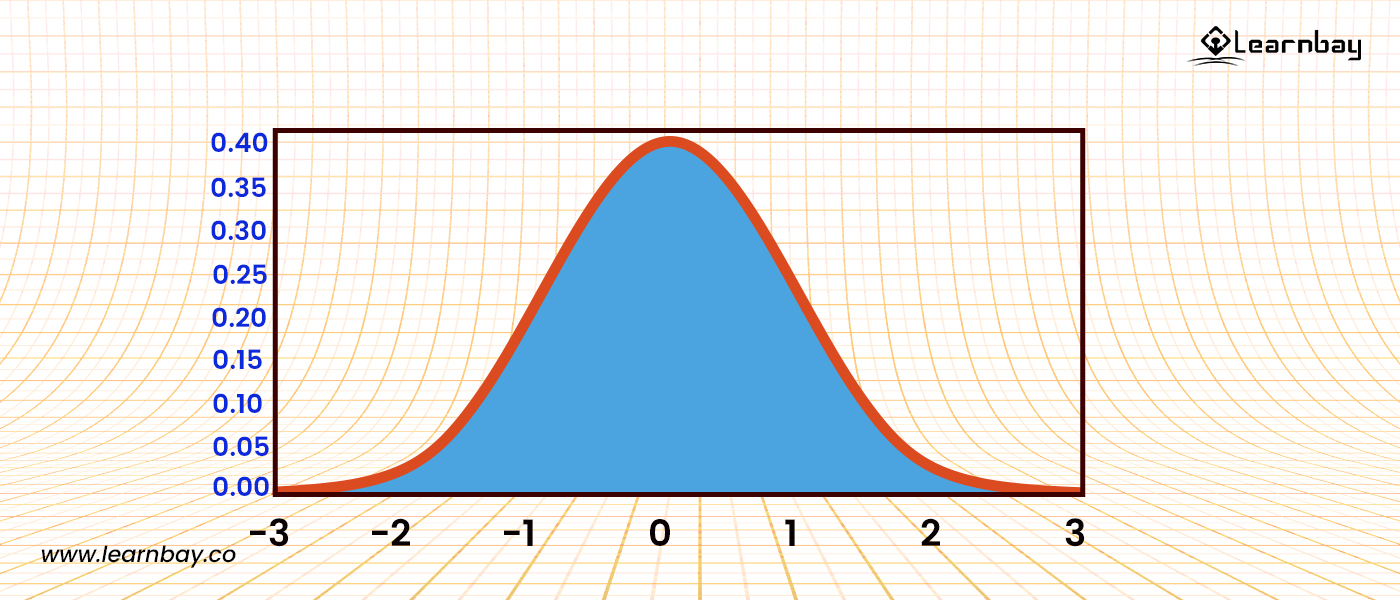# Normal and Gaussian Distribution

By Manas Kochar Category Machine Learning Reading time 3-4 mins Published on Mar 29, 2022

Learn the Fundamental Concepts of Normal Distribution in Statistics

Statistics, the foundation of data Science, has a fundamental element known as a normal distribution. Normal Distribution is also called Gaussian Distribution, which is considered the highest significant continuous probability distribution in statistics and probability theory. Gaussian Distribution is a bell-shaped curve. Throughout Physical science & Economics, the Normal Distribution usually nearly or perfectly represents various random variables.

• Estimating other probability distributions is one of the main functions of normal distributions. It is used for mean, median, and mode for continuous values.

• It follows the normal Distribution with an equal number of measurements on the right and left sides of the mean value.

• The mean is situated at the center of the curve, the right values from the mean are greater than the mean value, and the left values from the mean are smaller than the mean.

You all know the basic meaning of mean, median, and mode. The mean is an average of the values, the median is the center value of the distribution, and the mode is the value of the distribution, which frequently occurs.

In normal distribution in statistics , the mean, median, and mode values are all the same. If the values generate skewness, then they are not normally distributed. Normal distribution statistics are very important because they fit many occurrences, such as heights, blood pressure, measurement errors, and numerical values.

## What is the normal distribution in statistics?

The density function of probability for a constant random variable inside a system defines the normal distribution in statistics.

Assuming that f(x) is the probability density function and X is a random variable, it provides a function integrated across the domain or interval (x to x+dx). The frequency of random variable X is given by taking values within x and x+dx into account.

## What are the main properties of normal distribution?

### 1. Standard Deviation of Normal Distribution:-

The Gaussian and normal distribution are the same in statistics theory. The normal distribution curve is made with the help of the probability density function with the random values. F(x) is the PDF function, and x is the value of Gaussian which is used to represent the real values of random variables having unknown distribution.

There is a property of Gaussian distribution, known as the Empirical formula, which shows the confidence interval the value comes under.

For example, the normal distribution in statistics contains a mean value of 0 and a standard deviation of 1.The empirical rule also referred to as the three-sigma rule or 68-95-99.7 rule, is a statistical rule which states that for a normal distribution, almost all data falls within three standard deviations (denoted by σ) of the mean (denoted by µ).

Broken down,

• The empirical rule shows that 68% falls within the first standard deviation (µ ± σ),

• 95% within the first two standard deviations (µ ± 2σ),

• And 99.7% within the first three standard deviations (µ ± 3σ).

#### Python code for plotting the normal distribution graph:-

• import matplotlib.pyplot as plt

• import NumPy as np

• import Scipy.stats as stats

• import math

• mu = 0

• variance = 1

• sigma = math.sqrt(variance)

• x = np.linspace(mu - 3sigma, mu + 3sigma, 100)

• plt.plot(x, stats.norm.pdf(x, mu, sigma))

• show()The above code shows the Gaussian Distribution with a 99% of confidence interval, a standard deviation of 3, and a mean of 0.

### 2. Skewness and Kurtosis:-

Skewness means the degree up to which the symmetric distribution is maintained. When the left side tail is longer than the right side, the data set's distribution has a negative skewness (less than zero). And if the right side tail is longer, the skewness is positive.

As Normal Distribution is symmetric, it shows zero skewness.

Kurtosis measures the width of the distribution's tail end concerning its tails. Distributions with kurtosis above 3.0 have tail data that exceeds normal distribution tails. This extra kurtosis is statistically characterized as leptokurtic, although it is more popularly called "fat tails". This presence of fat tails in a financial market scenario is called tail risk.

The normal distribution shows a 3.0 kurtosis level.

#### Note:

Why Is the Normal Distribution Called "Normal"?

When the statisticians started doing the equations, they noticed similar shapes regularly occurring for different equations. By seeing this, they thought it appropriate to coin the term "normal" for the distribution.

In addition, since the distribution was seen over and over as common or typical, the name "normal" came to be attached to the distribution. At the beginning of the 20th century, the term became popular with this type of distribution.

## How is the normal distribution used in finance?

• Traders can use standard deviations to suggest suitable trades. However, this trading is often done in extremely short periods because bigger periods make picking entry and exit spots considerably more difficult.

• Asset pricing and price action both utilize the concept of a normal distribution in statistics. We may plot price points over time to match recent price behavior into a normal distribution. In this situation, the more a price movement deviates from the mean, the more likely a given asset is either under or overpriced.

• In the same way, many statistical theories run under the premise of following a normal distribution and model their asset pricing after that. The price fluctuations usually have a bigger kurtosis (>3), showing fat tails.

• Price fluctuations of over three standard deviations outside the mean had already occurred more frequently than predicted under a normal distribution. Whether an asset has followed a normal distribution for long, there is no guarantee that previous performance informs future possibilities.

## What are the limitations of normal distribution in finance?

The normal distribution has some constraints when it comes to financing, as its implementations in finance are restricted, like the expected stock market returns, which are not supported by the normal distribution in statistics.

• Prices generally adhere to a more right-skewed log-normal distribution having wider tails. As a result, depending excessively on a bell curve for forecasting these occurrences might provide incorrect results.

• It's hard for most analysts to solve this problem as the statistical distribution to be used is often unknown.